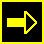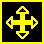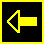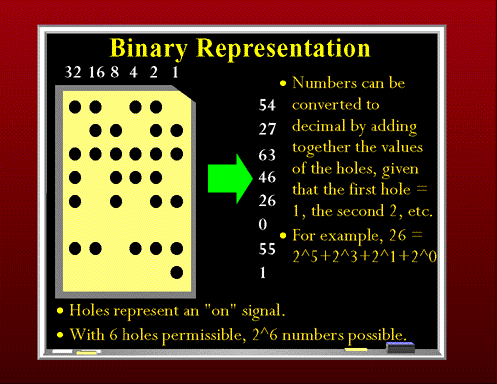Binary RepresentationNextIndexPrevHollerith's machine though had limitations. It was strictly limited to tabulation. The punched cards could not be used to direct more complex computations. In 1941, Konrad Zuse(*), a German who had developed a number of calculating machines, released the first programmable computer designed to solve complex engineering equations. The machine, called the Z3, was controlled by perforated strips of discarded movie film. As well as being controllable by these celluloid strips, it was also the first machine to work on the binary system, as opposed to the more familiar decimal system.

The binary system is composed of 0s and 1s. A punch card with its two states--a hole or no hole-- was admirably suited to representing things in binary. If a hole was read by the card reader, it was considered to be a 1. If no hole was present in a column, a zero was appended to the current number. The total number of possible numbers can be calculated by putting 2 to the power of the number of bits in the binary number. A bit is simply a single occurrence of a binary number--a 0 or a 1. Thus, if you had a possible binary number of 6 bits, 64 different numbers could be generated. (2^(n-1))

Binary representation was going to prove important in the future design of computers which took advantage of a multitude of two-state devices such card readers, electric circuits which could be on or off, and vacuum tubes.

* Zuse died in January of 1996.

Computers: From the Past to the Present